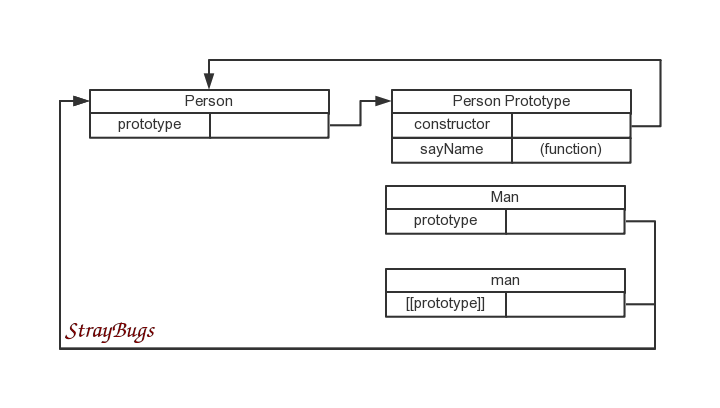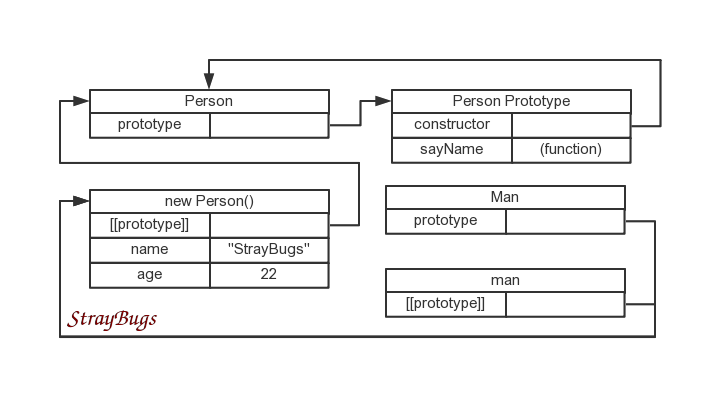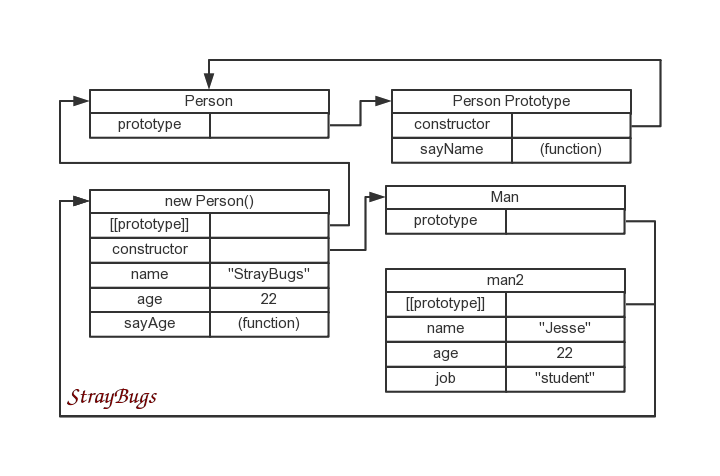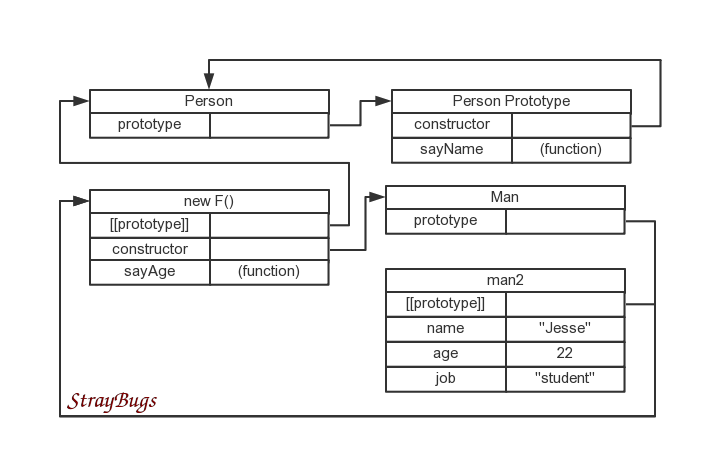# JavaScript 继承总结

``````function Person(name) {
if(name !== undefined) {
this.name = name;
} else {
this.name = "StrayBugs";
}
this.age = 22;
}

Person.prototype.sayName = function() {
};``````

## 原型链

JavaScript 中实现继承第一个方法是利用原型链。

``````function Man() {

}

//第一种
Man.prototype = Person;
var man = new Man();

//第二种
Man.prototype = Person();
var man = new Man();

//第三种
Man.prototype = new Person();
var man = new Man();``````## 借用构造函数

``````function Man(name) {

//每个实例都可以有自己的名字
Person.call(this, name);

//子类增加的属性
this.job = 'student';
}

var man1 = new Man();
var man2 = new Man('Jesse');

## 组合继承

``````function Man(name) {

//每个实例都可以有自己的名字
Person.call(this, name);

//子类增加的属性
this.job = 'student';
}

//继承方法
Man.prototype = new Person();
Man.prototype.constructor = Man;

//子类增加的方法
Man.prototype.sayAge = function() {
};

var man1 = new Man();
var man2 = new Man('Jesse');

man1.sayName(); //"StrayBugs"
man2.sayName(); //"Jesse"
man2.sayAge();  //22## 原型式继承

``````function object(o) {
function F() {}
F.prototype = o;
return new F();
}``````

``````var person = new Person();

var man = object(person);
man.name = 'Jesse';

man.sayName(); //"Jesse"

person.name = 'StrayBugs';
person.age = 101;

man.sayName(); //"Jesse"

ECMAScript5 新增 `Object.create()` 方法规范化了原型式继承。

## 寄生式继承

``````function createMan(person) {

//这里不一定是 object()，可以是任意能返回新对象的函数
var man = object(person);
man.sayAge = function() {
};
return man;
}

var person = new Person();

var man = createMan(person);
man.sayAge(); //22``````

## 寄生组合式继承

``````function inheritPrototype(Man, Person) {
//只复制原型
var p = object(Person.prototype);
p.constructor = Man;
Man.prototype = p;
}``````

``````function Man(name) {
Person.call(this, name);
this.job = 'student';
}

inheritPrototype(Man, Person);

Man.prototype.sayAge = function() {
};``````

``````var man = new Man('Jesse');
man.sayAge();  //22